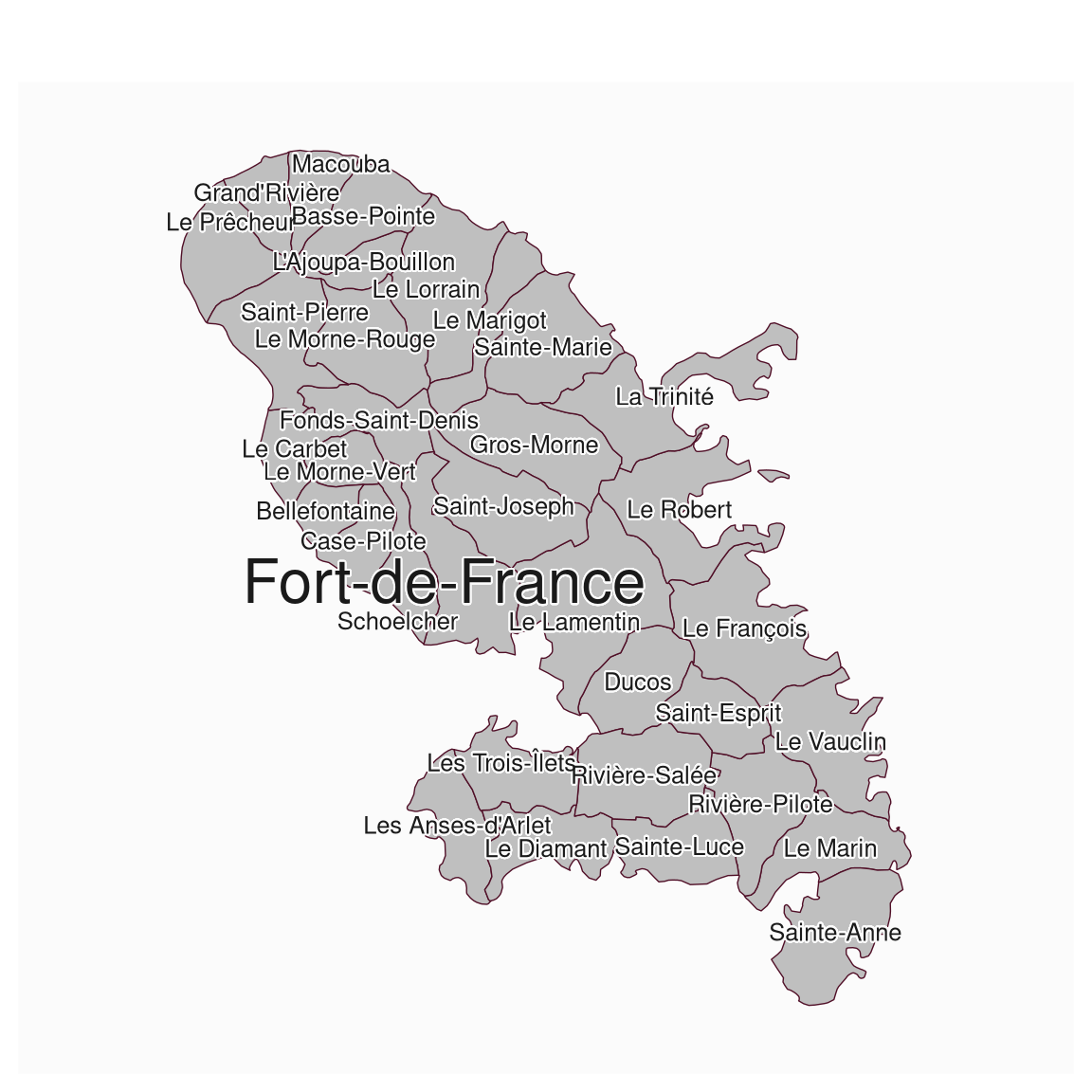Put labels on a map.

mf_label(
x,
var,
col,
cex = 0.7,
overlap = TRUE,
lines = TRUE,
halo = FALSE,
bg,
r = 0.1,
...
)

## Arguments

x

object of class sf

var

name(s) of the variable(s) to plot

col

labels color

cex

labels cex

overlap

if FALSE, labels are moved so they do not overlap.

lines

if TRUE, then lines are plotted between x,y and the word, for those words not covering their x,y coordinate

halo

if TRUE, a 'halo' is displayed around the text and additional arguments bg and r can be modified to set the color and width of the halo.

bg

halo color

r

width of the halo

...

further text arguments.

## Value

No return value, labels are displayed.

## Examples

mtq <- mf_get_mtq()
mf_map(mtq)
mf_label(
x = mtq, var = "LIBGEO", halo = TRUE, cex = 0.8,
overlap = FALSE, lines = FALSE
)